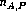Next: Testing Individual Performance Up: Testing Team Performance Previous: The Permutation Test

# Computation

In order to simplify the computation of the permutation test, we implemented a small C program to do the job. Below is a brief explanation of the algorithm applied in the program (given two documents A and B, and certain numbers of subjects):

1. Compute an array of ``masks'', which are binary numbers with (+) digits. We require the ``cross sum'' of the mask to be. A mask determines a particular dillution of the groups of people. We will havedifferent masks, and thus this is the number of possible permutations of the subjects.

2. Each subjects is associated with a digit in the mask. For document A, a mask digit 1 means that the subject is considered a PBR subject, while a mask digit 0 means he is considered a NASA subject - regardless of what technique he actually used. For document B the mask digit takes the opposite meaning.

3. For each of the masks, the perspectives are manipulated accordingly, and then all the possible teams are combined. The test statistic is computed as described above.

4. The various permutations are ranked according to the test statistic. The corresponding degree of dillution is indicated, and the significance of the test is determined by locating the percentile of the non-dilluted permutation.

Generated by latex2html-95.1
Mon Jun 24 13:58:35 EDT 1996

Web Accessibility